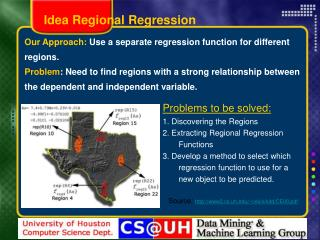DownloadDownload PresentationOur Approach: Use a separate regression function for different regions.

# Our Approach: Use a separate regression function for different regions.

Télécharger la présentation## Our Approach: Use a separate regression function for different regions.

- - - - - - - - - - - - - - - - - - - - - - - - - - - E N D - - - - - - - - - - - - - - - - - - - - - - - - - - -
##### Presentation Transcript

1. Idea Regional Regression Our Approach: Use a separate regression function for different regions. Problem: Need to find regions with a strong relationship between the dependent and independent variable. Problems to be solved: 1. Discovering the Regions 2. Extracting Regional Regression Functions 3. Develop a method to select which regression function to use for a new object to be predicted. Source: http://www2.cs.uh.edu/~ceick/kdd/CE09.pdf

2. Motivation Regional Knowledge & Coefficient Estimates • In geo-referenced dataset, most relationships only exist at regional level but not at the global level. • 1st law of geography: “Everything is related to everything else but nearby things are more related than distant things” (Tobler) • Coefficient estimates in geo-referenced datasets spatially vary  we need regression methods to discover regional coefficient estimates that captures underlyingstructure of data. • Using human-made boundaries (zip code etc.) is not good idea since they do not reflect patterns in spatially variance.

3. Motivation Geo-Regression Analysis Methods • Regression Trees • Data is split in a top-down approach using a greedy algorithm; uses constants as regression functions • Discovers only rectangular shapes • Geographically Weighted Regression (GWR) • an instance-based, local spatial statistical technique used to analyze spatial non-stationarity. • generates a separate regression for each possible query point “online”determined using a grid or kernel • a weight assigned to each observation that is based on its distance to the query point.

4. Motivation Example 1: Why We Need Regional Knowledge? Arsenic Fluoride Regression Result: A positive linear regression line (Arsenic increases with increasing Fluoride concentration)

5. Motivation Example 1: Why We Need Regional Knowledge? Location 1 Location 2 Arsenic Fluoride • A negative linear Regression line in both locations (Arsenic decreases with increasing Fluoride concentration) • A reflection of Simpson’s paradox.

6. Motivation Example 2: Houston House Price Estimate • Dependent variable: House_Price • Independent variables: noOfRooms, squareFootage, yearBuilt, havePool, attachedGarage, etc..

7. Motivation Example 2: Houston House Price Estimate • Global Regression (OLS) produces the coefficient estimates, R2 value, and error etc.. a model • This model assumes all areas have same coefficients • E.g. attribute havePool has a coefficient of +9,000 • (~having a pool adds \$9,000 to a house price) • In reality this changes. A house of \$100K and a house of \$500K or different zip codes or locations. • Having a pool in a house in luxury areasis very different (~\$40K) than having a pool in a house in Suburbs(~\$5K).

8. Motivation Example 2: Houston House Price Estimate • Solution: To apply local regression to each zip code • produces 50+ sets of parameter estimates • it captures spatial variations in the relationship better than global model • But it is very naïve and has problems • there is spatial variation within zip codes • assumes discontinuity but most spatial patterns are continuous and they do not stop & start at the border.

9. Motivation Example 2: Houston House Price Estimate \$350,000 \$180,000 • Houses A, B have very similar characteristics • OLS produces single parameter estimates for predictor variables like noOfRooms, squareFootage, yearBuilt, etc

10. Motivation Example 2: Houston House Price Estimate • If we use zip code as regions, they are in same region • If we use a grid structure • They are in different regions but some houses similar to B (lake view) are in same region with A and this will effect coefficient estimate • More importantly, the house around U-shape lake show similar pattern and should be in the same region, we miss important information.